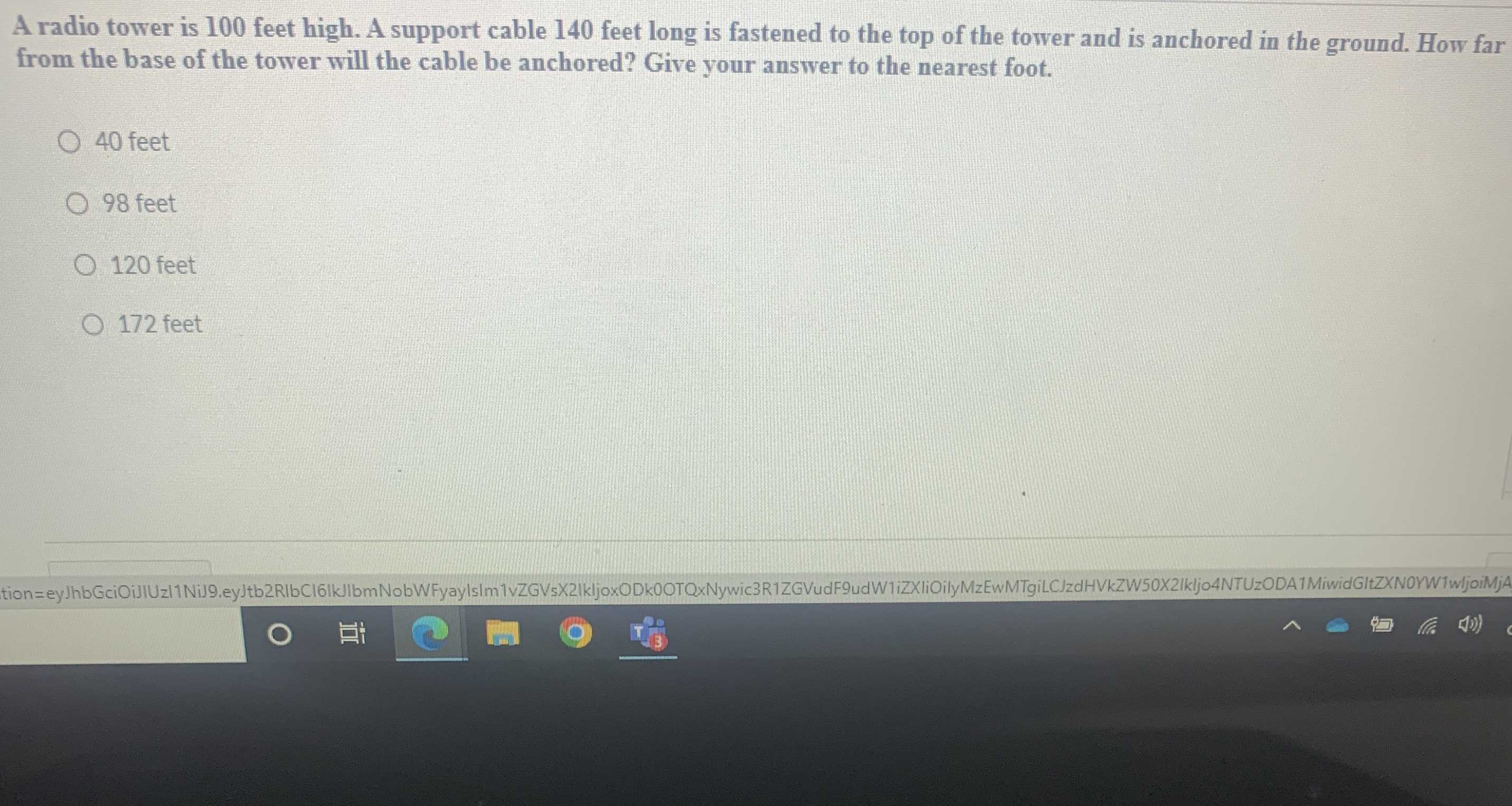### Still have math questions?

Trigonometry
QuestionA radio tower is $$100$$ feet high. A support cable $$140$$ feet long is fastened to the top of the tower and is anchored in the ground. How far from the base of the tower will the cable be anchored? Give your answer to the nearest foot.

$$40$$ feet

$$98$$ feet

$$120$$ feet

$$172$$ feet